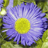# Select By Query from Items in Python List

10131
3
10-10-2016 12:53 PMbyMVP Regular Contributor

I'd like to create a SQL query statement which can select by multiple items in a Python string.

Can this be done?

``````lyr = r''
my_list = ['12','13','14','15']

for i in my_list:
qry = "OBJECTID" + i

arcpy.SelectLayerByAttribute_management(lyr, qry)‍‍‍‍‍‍‍``````

The problem I'm having is formatting the list properly so it won't trip up the SQL statement.  Should I create a new list with the items separated by a "+" character?

Tags (3)
1 Solution

Accepted SolutionsNew Contributor

Have you tried using the SQL "IN" Operator?

SQL IN Operator

http://www.w3schools.com/sql/sql_in.asp

``````# Path to the Layer
lyr = r"<NAME_OF_LAYER>"

# Integer list of OBJECTID(s)
oid = [12, 13, 14, 15]

# SQL Query
sql = "{} IN ({})".format("OBJECTID", ", ".join([str(n) for n in oid]))

# Tool Execution
arcpy.management.SelectLayerByAttribute(lyr, "NEW_SELECTION", sql)‍‍‍‍‍‍‍‍‍‍‍‍‍‍‍‍‍‍‍‍‍‍‍‍‍‍‍‍‍‍‍‍‍``````

The above format and list comprehension would yield the following string:

'OBJECTID IN (12, 13, 14, 15)'

The trick to this is that you need to create a string that represents valid SQL Syntax. This can be done is quite a few ways. I've listed a few quick options below.

``````# A few possible SQL Queries
sql = " OR ".join(["OBJECTID = {}".format(n) for n in oid])
# => OBJECTID = 12 OR OBJECTID = 13 OR OBJECTID = 14 OR OBJECTID = 15

sql = "{0} >= {1} AND {0} <= {2}".format("OBJECTID", min(oid), max(oid))
# => OBJECTID >= 12 AND OBJECTID <= 15

sql = "{} BETWEEN {} AND {}".format("OBJECTID", min(oid), max(oid))
# => OBJECTID BETWEEN 12 AND 15‍‍‍‍‍‍‍‍‍``````
3 RepliesNew Contributor

Have you tried using the SQL "IN" Operator?

SQL IN Operator

http://www.w3schools.com/sql/sql_in.asp

``````# Path to the Layer
lyr = r"<NAME_OF_LAYER>"

# Integer list of OBJECTID(s)
oid = [12, 13, 14, 15]

# SQL Query
sql = "{} IN ({})".format("OBJECTID", ", ".join([str(n) for n in oid]))

# Tool Execution
arcpy.management.SelectLayerByAttribute(lyr, "NEW_SELECTION", sql)‍‍‍‍‍‍‍‍‍‍‍‍‍‍‍‍‍‍‍‍‍‍‍‍‍‍‍‍‍‍‍‍‍``````

The above format and list comprehension would yield the following string:

'OBJECTID IN (12, 13, 14, 15)'

The trick to this is that you need to create a string that represents valid SQL Syntax. This can be done is quite a few ways. I've listed a few quick options below.

``````# A few possible SQL Queries
sql = " OR ".join(["OBJECTID = {}".format(n) for n in oid])
# => OBJECTID = 12 OR OBJECTID = 13 OR OBJECTID = 14 OR OBJECTID = 15

sql = "{0} >= {1} AND {0} <= {2}".format("OBJECTID", min(oid), max(oid))
# => OBJECTID >= 12 AND OBJECTID <= 15

sql = "{} BETWEEN {} AND {}".format("OBJECTID", min(oid), max(oid))
# => OBJECTID BETWEEN 12 AND 15‍‍‍‍‍‍‍‍‍``````byMVP Regular Contributor